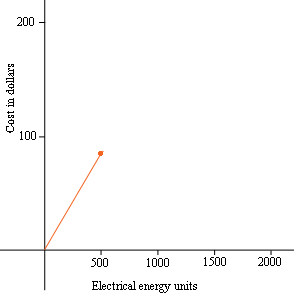SEARCH HOMEMath Central Quandaries & QueriesQuestion from Zoe, a student: Lightning Energy charges residential users for each unit of electrical energy bought from them each quarter, according to the scale below: 0-500 units cost 17 cents per unit 500-1500 units cost $\$85$plus 10 cents for each unit in the excess of 500 1500 or more units cost$\$185$ plus 9 cents for each unit in excess of 1500 Sketch a graph showing the charge C as a function of U for up to 2000 electricity unitsHi Zoe,

I set up a coordinate system with electrical energy units on the horizontal axis and cost on the vertical axis. Here is how I would progress with the first leg of the graph.

The graph starts at (0, 0), 0 units of energy cost $\$0.$The energy costs$\$0.17$ for each unit used up to 500 units. How much will 500 units cost? Place a point on the graph to represent the cost a of 500 units and join this point to (0, 0) by a straight line.Can you continue from here?

Penny* Registered trade mark of Imperial Oil Limited. Used under license.Math Central is supported by the University of Regina and the Imperial Oil Foundation.